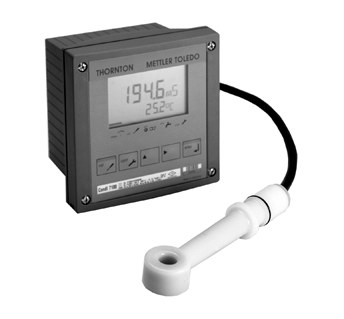•• # Siemens (Unit of Electrical Conductance)

The siemens is the standard unit of electrical conductance. It is the inverse of resistance and is equal to one divided by resistance, or current divided by voltage. One siemens is equal to one ampere per volt.## History of the Siemens

The siemens was defined at an international conference in 1881, and is named after Ernst Werner von Siemens (1816-1892), a German inventor. The symbol for Siemens is a capital S. The previous unit for electrical conductance was called the mho, and it is still used today in some areas of electronics. The name mho is actually ohm written backwards, signifying the inverse relationship between conductance and resistance.

The siemens was originally a unit of resistance, and had a value slightly less than one ohm. It was based on a mercury standard, and unrelated to voltage or current which made calculations difficult. This contributed to the decision to make the ohm the standard unit of resistance in 1881.

## Measuring Siemens

The electrical conductance of a material can be calculated from it's resistance. For example, the conductance of a one ohm resistor is one siemens, while the conductance of a hundred ohm resistor is one hundredth of a siemens.

478 queries in 0.586 seconds.